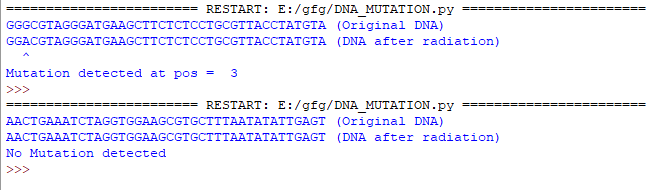Related Articles

# Detect Mutation using Python

• Last Updated : 12 Nov, 2020

Prerequisite: Random Numbers in Python

The following article depicts how Python can be used to detect a mutated DNA strand.

Attention geek! Strengthen your foundations with the Python Programming Foundation Course and learn the basics.

To begin with, your interview preparations Enhance your Data Structures concepts with the Python DS Course. And to begin with your Machine Learning Journey, join the Machine Learning - Basic Level Course

### Functions Used

1. generateDNASequence(): This method generates a random DNA strand of length 40 characters using the list of DNA bases A,C,G,T. This method returns the generated DNA strand.
2. applyGammaRadiation(): This method takes the DNA strand generated by the above method as input parameter and then alters the strand at a random position only if the probability of mutation, which is also generated randomly is above 50%. The DNA base at the chosen position must be different from the DNA base with which it is replaced. This method returns the altered DNA strand.
3. detectMutation(): This method takes the original and altered DNA strand as input and checks if the two strings are altered. If the strings are altered it returns the position of the altered DNA base

### Getting Started

The following steps are followed in order to achieve our required functionality:

• Import random library
• The generateDNASequence() function is used to generate DNA strands. DNA strand is generated by randomly choosing characters from the list of available options. When the string length becomes 40 the loop is completed and the DNA strand is returned.
• The applyGammaRadiation() function to alter DNA strands. The possibility of mutation is generated randomly. If the possibility of mutation generated randomly is greater than 50 then mutation occurs else mutation does not occur. If mutation were to occur, the position of mutation is chosen randomly.
• Next, the characters in DNA strand is converted to list.
• The character at the position of mutation is fetched. Since the fetched character should be different from the one replacing it, we remove the fetched character from the list of available choices for choosing another character in its place. The new character or DNA base is chosen from the list.
• Original DNA strand characters are again appended to a new list. The new base/character is set in the mutated position.
• The characters in the cl list is converted to string again using join(). This is the new mutated DNA string.
• If no mutation occurs, original dna is same as mutated DNA.
• Finally the mutated/unmutated DNA is returned.
• Then detectMutation() function is used to detect mutation. In this function, x and y take each character in dna and cdna for character by character comparison. If the character at the same index match, then the count is increased. Incase of mismatch the loop is broken.
• The count value points to the index before the position of mutation. If count=40 it means all the characters of the 2 strands match, hence no mutation If count is less than 40, it means mutation has occurred.

Below is the Implementation.

## Python3

 `# import random library``import` `random`` ` `# function to generate dna strands``def` `generateDNASequence():``   ` `    ``# list of available DNA bases``    ``l ``=` `[``'C'``, ``'A'``, ``'G'``, ``'T'``]``    ``res ``=` `""``    ``for` `i ``in` `range``(``0``, ``40``):``        ``# creating the DNA strand by appending ``        ``# random characters from the list``        ``res ``=` `res ``+` `random.choice(l)``    ``return` `res`` ` `# function to alter dna strands``def` `applyGammaRadiation(dna):``   ` `    ``# possibility of mutation is generated randomly``    ``pos ``=` `random.randint(``1``, ``100``)``    ``cdna ``=` `''``     ` `    ``# list of available DNA bases``    ``l ``=` `[``'C'``, ``'A'``, ``'G'``, ``'T'``]``     ` `    ``# if the possibility of mutation generated randomly``    ``# is >50 then mutation happens``    ``if``(pos > ``50``):``       ` `        ``# the position where mutation will take place``        ``# is chosen randomly``        ``changepos ``=` `random.randint(``0``, ``39``)``        ``dl ``=` `[]``         ` `        ``# the characters in DNA strand is converted to list``        ``dl[:``0``] ``=` `dna``         ` `        ``# the character at the determined mutation position ``        ``# is fetched.``        ``ch ``=` `"" ``+` `dl[changepos]``         ` `        ``# since the fetched character should be different from ``        ``# the one replacing it we remove the fetched character``        ``# from the list of available choices for choosing another``        ``# character in its place``        ``l.remove(ch)``         ` `        ``# the new character or DNA base is chosen from the list``        ``ms ``=` `random.choice(l)``        ``cl ``=` `[]``         ` `        ``# DNA strand characters are again appended to a new list``        ``cl[:``0``] ``=` `dna``         ` `        ``# the new base in the mutated position is set``        ``cl[changepos] ``=` `ms``         ` `        ``# the characters in the cl list is converted to string again``        ``# this is the new mutated DNA string``        ``cdna ``=` `''.join([``str``(e) ``for` `e ``in` `cl])``     ` `    ``# if possibility of mutation is less than 50% then no ``    ``# mutation happens``    ``else``:``         ` `        ``# if no mutation occurs original dna is same as mutated dna``        ``cdna ``=` `dna``    ``return` `cdna`` ` `# function to detect mutation``def` `detectMutation(dna, cdna):``    ``count ``=` `0``     ` `    ``# x and y take each character in dna and cdna``    ``# for character by character comparison``    ``for` `x, y ``in` `zip``(dna, cdna):``        ` `        ``# if the character at the same index match``        ``# then the count is increased``        ``if` `x ``=``=` `y:``            ``count ``=` `count ``+` `1``         ` `        ``# incase of mismatch the loop is broken``        ``else``:``            ``break`` ` `    ``# the count value points to the index before the ``    ``# position of mutation``    ``return` `count`` ` ` ` `dna ``=` `generateDNASequence()``print``(dna``+``" (Original DNA)"``)``cdna ``=` `applyGammaRadiation(dna)``print``(cdna``+``" (DNA after radiation)"``)``count ``=` `detectMutation(dna, cdna)`` ` `# if count=40 it means all the characters of the 2 strands match``# hence no mutation``if` `count ``=``=` `40``:``    ``print``(``"No Mutation detected"``)`` ` `# if count is less than 40``# it means mutation has occurred``else``:``     ` `    ``# ^ denotes the position of mutation``    ``pos ``=` `"^"``    ``print``(pos.rjust(count``+``1``))``    ``print``(``"Mutation detected at pos = "``, (count``+``1``))`

OutputMy Personal Notes arrow_drop_up